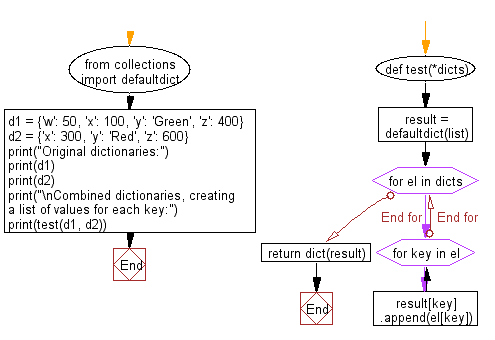﻿ Python: Combine two or more dictionaries, creating a list of values for each key - w3resource# Python: Combine two or more dictionaries, creating a list of values for each key

## Python dictionary: Exercise-68 with Solution

Write a Python program to combines two or more dictionaries, creating a list of values for each key.

• Create a new collections.defaultdict with list as the default value for each key and loop over dicts.
• Use dict.append() to map the values of the dictionary to keys.
• Use dict() to convert the collections.defaultdict to a regular dictionary.

Sample Solution:

Python Code:

``````from collections import defaultdict
def test(*dicts):
result = defaultdict(list)
for el in dicts:
for key in el:
result[key].append(el[key])
return dict(result)

d1 = {'w': 50, 'x': 100, 'y': 'Green', 'z': 400}
d2 = {'x': 300, 'y': 'Red', 'z': 600}

print("Original dictionaries:")
print(d1)
print(d2)
print("\nCombined dictionaries, creating a list of values for each key:")
print(test(d1, d2))
```
```

Sample Output:

```Original dictionaries:
{'w': 50, 'x': 100, 'y': 'Green', 'z': 400}
{'x': 300, 'y': 'Red', 'z': 600}

Combined dictionaries, creating a list of values for each key:
{'w': , 'x': [100, 300], 'y': ['Green', 'Red'], 'z': [400, 600]}
```

Flowchart:## Visualize Python code execution:

The following tool visualize what the computer is doing step-by-step as it executes the said program:

Python Code Editor:

Have another way to solve this solution? Contribute your code (and comments) through Disqus.

What is the difficulty level of this exercise?

Test your Programming skills with w3resource's quiz.

﻿

## Python: Tips of the Day

Decapitalizes the first letter of a string:

Example:

```def tips_decapitalize(s, upper_rest=False):
return s[:1].lower() + (s[1:].upper() if upper_rest else s[1:])
print(tips_decapitalize('PythonTips'))
print(tips_decapitalize('PythonTips', True))
```

Output:

```pythonTips
pYTHONTIPS
```